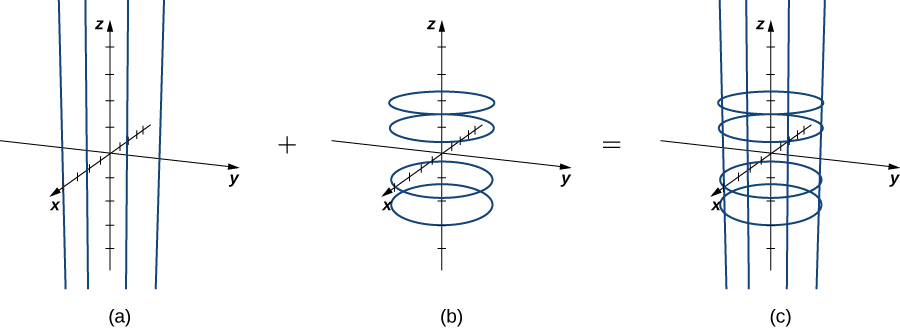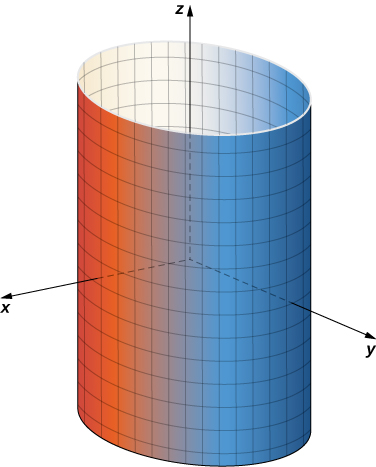# 6.6 Surface integrals

 Page 1 / 27
• Find the parametric representations of a cylinder, a cone, and a sphere.
• Describe the surface integral of a scalar-valued function over a parametric surface.
• Use a surface integral to calculate the area of a given surface.
• Explain the meaning of an oriented surface, giving an example.
• Describe the surface integral of a vector field.
• Use surface integrals to solve applied problems.

We have seen that a line integral is an integral over a path in a plane or in space. However, if we wish to integrate over a surface (a two-dimensional object) rather than a path (a one-dimensional object) in space, then we need a new kind of integral that can handle integration over objects in higher dimensions. We can extend the concept of a line integral to a surface integral to allow us to perform this integration.

Surface integrals are important for the same reasons that line integrals are important. They have many applications to physics and engineering, and they allow us to develop higher dimensional versions of the Fundamental Theorem of Calculus. In particular, surface integrals allow us to generalize Green’s theorem to higher dimensions, and they appear in some important theorems we discuss in later sections.

## Parametric surfaces

A surface integral is similar to a line integral, except the integration is done over a surface rather than a path. In this sense, surface integrals expand on our study of line integrals. Just as with line integrals, there are two kinds of surface integrals: a surface integral of a scalar-valued function and a surface integral of a vector field.

However, before we can integrate over a surface, we need to consider the surface itself. Recall that to calculate a scalar or vector line integral over curve C , we first need to parameterize C . In a similar way, to calculate a surface integral over surface S , we need to parameterize S . That is, we need a working concept of a parameterized surface (or a parametric surface ), in the same way that we already have a concept of a parameterized curve.

A parameterized surface is given by a description of the form

$\text{r}\left(u,v\right)=⟨x\left(u,v\right),y\left(u,v\right),z\left(u,v\right)⟩.$

Notice that this parameterization involves two parameters, u and v , because a surface is two-dimensional, and therefore two variables are needed to trace out the surface. The parameters u and v vary over a region called the parameter domain, or parameter space —the set of points in the uv -plane that can be substituted into r . Each choice of u and v in the parameter domain gives a point on the surface, just as each choice of a parameter t gives a point on a parameterized curve. The entire surface is created by making all possible choices of u and v over the parameter domain.

## Definition

Given a parameterization of surface $\text{r}\left(u,v\right)=⟨x\left(u,v\right),y\left(u,v\right),z\left(u,v\right)⟩,$ the parameter domain of the parameterization is the set of points in the uv -plane that can be substituted into r .

## Parameterizing a cylinder

Describe surface S parameterized by

$\text{r}\left(u,v\right)=⟨\text{cos}\phantom{\rule{0.2em}{0ex}}u,\text{sin}\phantom{\rule{0.2em}{0ex}}u,v⟩,\text{−}\infty

To get an idea of the shape of the surface, we first plot some points. Since the parameter domain is all of ${ℝ}^{2},$ we can choose any value for u and v and plot the corresponding point. If $u=v=0,$ then $\text{r}\left(0,0\right)=⟨1,0,0⟩,$ so point (1, 0, 0) is on S . Similarly, points $\text{r}\left(\pi ,2\right)=\left(-1,0,2\right)$ and $\text{r}\left(\frac{\pi }{2},4\right)=\left(0,1,4\right)$ are on S .

Although plotting points may give us an idea of the shape of the surface, we usually need quite a few points to see the shape. Since it is time-consuming to plot dozens or hundreds of points, we use another strategy. To visualize S , we visualize two families of curves that lie on S. In the first family of curves we hold u constant; in the second family of curves we hold v constant. This allows us to build a “skeleton” of the surface, thereby getting an idea of its shape.

First, suppose that u is a constant K . Then the curve traced out by the parameterization is $⟨\text{cos}\phantom{\rule{0.2em}{0ex}}K,\text{sin}\phantom{\rule{0.2em}{0ex}}K,v⟩,$ which gives a vertical line that goes through point $\left(\text{cos}\phantom{\rule{0.2em}{0ex}}K,\text{sin}\phantom{\rule{0.2em}{0ex}}K,v\right)$ in the xy -plane.

Now suppose that v is a constant K. Then the curve traced out by the parameterization is $⟨\text{cos}\phantom{\rule{0.2em}{0ex}}u,\text{sin}\phantom{\rule{0.2em}{0ex}}u,K⟩,$ which gives a circle in plane $z=K$ with radius 1 and center (0, 0, K ).

If u is held constant, then we get vertical lines; if v is held constant, then we get circles of radius 1 centered around the vertical line that goes through the origin. Therefore the surface traced out by the parameterization is cylinder ${x}^{2}+{y}^{2}=1$ ( [link] ).(a) Lines ⟨ cos K , sin K , v ⟩ for K = 0 , π 2 , π , and 3 π 2 . (b) Circles ⟨ cos u , sin u , K ⟩ for K = −2 , −1 , 1 , and 2 . (c) The lines and circles together. As u and v vary, they describe a cylinder.

Notice that if $x=\text{cos}\phantom{\rule{0.2em}{0ex}}u$ and $y=\text{sin}\phantom{\rule{0.2em}{0ex}}u,$ then ${x}^{2}+{y}^{2}=1,$ so points from S do indeed lie on the cylinder. Conversely, each point on the cylinder is contained in some circle $⟨\text{cos}\phantom{\rule{0.2em}{0ex}}u,\text{sin}\phantom{\rule{0.2em}{0ex}}u,k⟩$ for some k , and therefore each point on the cylinder is contained in the parameterized surface ( [link] ).Cylinder x 2 + y 2 = r 2 has parameterization r ( u , v ) = ⟨ r cos u , r sin u , v ⟩ , 0 ≤ u ≤ 2 π , − ∞ < v < ∞ .

where we get a research paper on Nano chemistry....?
nanopartical of organic/inorganic / physical chemistry , pdf / thesis / review
Ali
what are the products of Nano chemistry?
There are lots of products of nano chemistry... Like nano coatings.....carbon fiber.. And lots of others..
learn
Even nanotechnology is pretty much all about chemistry... Its the chemistry on quantum or atomic level
learn
da
no nanotechnology is also a part of physics and maths it requires angle formulas and some pressure regarding concepts
Bhagvanji
hey
Giriraj
Preparation and Applications of Nanomaterial for Drug Delivery
revolt
da
Application of nanotechnology in medicine
what is variations in raman spectra for nanomaterials
I only see partial conversation and what's the question here!
what about nanotechnology for water purification
please someone correct me if I'm wrong but I think one can use nanoparticles, specially silver nanoparticles for water treatment.
Damian
yes that's correct
Professor
I think
Professor
Nasa has use it in the 60's, copper as water purification in the moon travel.
Alexandre
nanocopper obvius
Alexandre
what is the stm
is there industrial application of fullrenes. What is the method to prepare fullrene on large scale.?
Rafiq
industrial application...? mmm I think on the medical side as drug carrier, but you should go deeper on your research, I may be wrong
Damian
How we are making nano material?
what is a peer
What is meant by 'nano scale'?
What is STMs full form?
LITNING
scanning tunneling microscope
Sahil
how nano science is used for hydrophobicity
Santosh
Do u think that Graphene and Fullrene fiber can be used to make Air Plane body structure the lightest and strongest. Rafiq
Rafiq
what is differents between GO and RGO?
Mahi
what is simplest way to understand the applications of nano robots used to detect the cancer affected cell of human body.? How this robot is carried to required site of body cell.? what will be the carrier material and how can be detected that correct delivery of drug is done Rafiq
Rafiq
if virus is killing to make ARTIFICIAL DNA OF GRAPHENE FOR KILLED THE VIRUS .THIS IS OUR ASSUMPTION
Anam
analytical skills graphene is prepared to kill any type viruses .
Anam
Any one who tell me about Preparation and application of Nanomaterial for drug Delivery
Hafiz
what is Nano technology ?
write examples of Nano molecule?
Bob
The nanotechnology is as new science, to scale nanometric
brayan
nanotechnology is the study, desing, synthesis, manipulation and application of materials and functional systems through control of matter at nanoscale
Damian
Is there any normative that regulates the use of silver nanoparticles?
what king of growth are you checking .?
Renato
What fields keep nano created devices from performing or assimulating ? Magnetic fields ? Are do they assimilate ?
why we need to study biomolecules, molecular biology in nanotechnology?
?
Kyle
yes I'm doing my masters in nanotechnology, we are being studying all these domains as well..
why?
what school?
Kyle
biomolecules are e building blocks of every organics and inorganic materials.
Joe
can you provide the details of the parametric equations for the lines that defince doubly-ruled surfeces (huperbolids of one sheet and hyperbolic paraboloid). Can you explain each of the variables in the equations?By Richley CrapoBy Robert MurphyBy Jessica CollettBy John GabrieliBy Abby SharpBy OpenStaxBy OpenStaxBy RhodesBy Ann SchlosserBy Kevin Moquin